Next: SOLVING THE FREQUENCY-DEPENDENT EIKONAL Up: Biondi: Solving the frequency-dependent Previous: INTRODUCTION

# FREQUENCY-DEPENDENT EIKONAL

The frequency-dependent eikonal equation can be easily derived starting from the scalar wave equation:(1)
whereis the wavefield, as a function of the positionand time t, andis the slowness of the medium. Fourier transforming the time coordinate we get the Helmholtz equation: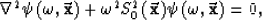(2)
whereis the transformed wavefield, and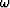the temporal frequency.

The next step is to substitute into the Helmholtz equation the trial solution,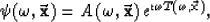(3)
where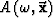is the amplitude of the wavefield, andthe time delay, or traveltime. Equating the real part of equation (2) we get the frequency-dependent eikonal equation, Beydoun (1985); Zhu (1988)(4)
whereis the phase slowness, that is function of frequency. Equating the imaginary part of equation (2) we get the transport equation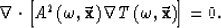(5)

The conventional eikonal equation is derived by dropping the second term on the right of equation (4). The second term can be dropped when(6)
that is, when the frequency of the propagating waves is sufficiently large, with respect to the relative amplitude variations. In this case, the traveltimes are independent from the amplitudes, and the two equations can be solved sequentially. First, the eikonal is solved for the traveltimes; second, the amplitudes are computed using the transport equation. The most common method for computing the amplitudes is to recognize that the transport equation dictates that the product of the square of the amplitude with the phase slowness (A2 S) is preserved along a ray tube. The amplitude can be thus expressed as(7)
where A0 is the amplitude at the source and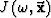is the Jacobian of mapping via rays Bleistein (1984). The amplitude is thus dependent on the phase slowness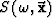,and on the Jacobian, which is proportional to the ray density. The validity of the condition expressed in (6) is thus dependent on both the rate of variation of the phase slowness and on the rate of variation of ray density. For example, in presence of caustics, the condition in (6) can be violated even if the phase-slowness function is smooth.Next: SOLVING THE FREQUENCY-DEPENDENT EIKONAL Up: Biondi: Solving the frequency-dependent Previous: INTRODUCTION
Stanford Exploration Project
11/18/1997